## Example Questions

### Example Question #8 : Rational Expressions

Simplify (4x)/(x– 4) * (x + 2)/(x– 2x)

x/(x – 2)2

(4x+ 8x)/(x+ 8x)

4/(x + 2)2

x/(x + 2)

4/(x – 2)2

4/(x – 2)2

Explanation:

Factor first.  The numerators will not factor, but the first denominator factors to (x – 2)(x + 2) and the second denomintaor factors to x(x – 2).  Multiplying fractions does not require common denominators, so now look for common factors to divide out.  There is a factor of x and a factor of (x + 2) that both divide out, leaving 4 in the numerator and two factors of (x – 2) in the denominator.

### Example Question #9 : Rational Expressions

what is 6/8 X 20/3

18/160
5
9/40
120/11
3/20
Explanation:

6/8 X 20/3 first step is to reduce 6/8 -> 3/4 (Divide top and bottom by 2)

3/4 X 20/3 (cross-cancel the threes and the 20 reduces to 5 and the 4 reduces to 1)

1/1 X 5/1 = 5

### Example Question #1 : How To Multiply Rational Expressions

Multiply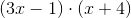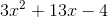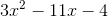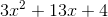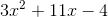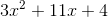Explanation:

When multiplying two binomials, it's best to remember the mnemonic FOIL, which stands for First, Outer, Inner, and Last. Going in the order of FOIL, we multiply the first term of each binomial, then the outer terms of the expression, then the inner terms of the expression, and finally the last term of each binomial. For this question we get: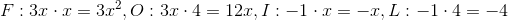Now that we have 'FOILed' our expression, we simply add our results to get the final answer.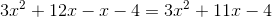### Example Question #2 : How To Multiply Rational Expressions

Express the following as a single rational expression, make sure you simplify your answer: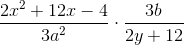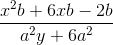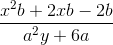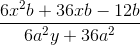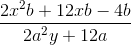Explanation:

To do this problem, simply multiply as you would with any fraction, combining like terms whenever possible:When multiplying, make sure to distribute through to all terms in the polynomial.Then you can factor out a six to simplify for your final answer:Tired of practice problems?

Try live online GRE prep today.